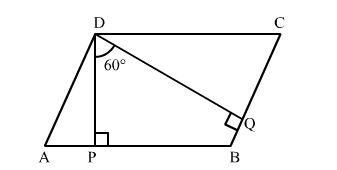# The angle between two altitudes of a parallelogram through the vertex of an obtuse angle of the parallelogram is 60°.

Question:

The angle between two altitudes of a parallelogram through the vertex of an obtuse angle of the parallelogram is 60°. Find the angles of the parallelogram.

Solution:Given: In parallelogram ABCDDP ABAQ  BC and ∠PDQ = 60°

In quadrilateral DPBQ, by angle sum property, we have

$\angle P D Q+\angle D P B+\angle B+\angle B Q D=360^{\circ}$

$\Rightarrow 60^{\circ}+90^{\circ}+\angle B+90^{\circ}=360^{\circ}$

$\Rightarrow \angle B=360^{\circ}-240^{\circ}$

$\Rightarrow \angle B=120^{\circ}$

Therefore, $\angle B=120^{\circ}$

Now,

$\angle B=\angle D=120^{\circ}$  (Opposite angles of a parallelogram are equal.)

$\angle A+\angle B=180^{\circ}$  (Adjacent angles of a parallelogram are supplementary.)

$\Rightarrow \angle A+120^{\circ}=180^{\circ}$

$\Rightarrow \angle A=180^{\circ}-120^{\circ}$

$\Rightarrow \angle A=60^{\circ}$

Also,

$\angle A=\angle C=60^{\circ}$    (Opposite angles of a parallleogram are equal.)

So, the angles of a parallelogram are $60^{\circ}, 120^{\circ}, 60^{\circ}$ and $120^{\circ}$.